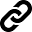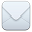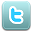SHARE:The data files that are provided on the Understanding America Study data pages provide cleaned versions of the raw data. Cleaned here means the followings:

• Answers given to questions that are not applicable anymore at survey completion (for example because a respondent went back in the survey and skipped over a previously answered question) are treated as if the questions were never asked. In the data files all questions that were asked, but not answered by the respondent are marked with ".e". All questions never seen by the respondent (or any dirty data) are marked with ".". The latter may mean that a respondent did not view the question because s/he skipped over it; or alternatively that s/he never reached that question in the survey due to a survey break off.
• For incomplete surveys the end date variables are set to ".c".
• Raw standard data variables are processed to match the standard variables and basic demographics listed here. As such, their names may differ from those in the survey codebook.

## Default variables

The mapping between the cleaned default data variables and the raw data is as follows:

Cleaned data variable Raw data variable
uasid Equivalent of prim_key.
primary_respondent

No equivalent. Is derived from sampletype as follows:

• primary_respondent=0 if sampletype in [3,6,8,17]
• otherwise primary_respondent=1
uashhid Equivalent of uashhid.
survhhid No equivalent. Calculated using cantor pairing of the uasid of all other members of the same UAS household at the time of the survey.
uasmembers No equivalent. Calculated as the sum of all other members of the same UAS household at the time of the survey.
sampletype

No equivalent. Is derived from sampletype as follows:

• sampletype=1 if sampletype in [1, 3, 7, 8, 10, 11, 12, 13, 14, 15, 16, 23, 26, 27, 28, 29, 30, 31, 32, 33]
• sampletype=2 if sampletype in [2, 4, 17]
• sampletype=3 if sampletype in [5, 6, 18, 19, 24, 25]
• sampletype=4 if sampletype in [20, 21, 22]
batch

No equivalent. Is derived from sampletype as follows:

• batch=1 if sampletype in [1, 3]
• batch=2 if sampletype in [2,17]
• batch=3 if sampletype=4
• batch=4 if sampletype in [5,6]
• batch=5 if sampletype in [7,8]
• batch=6 if sampletype=10
• batch=7 if sampletype=11
• batch=8 if sampletype=12
• batch=9 if sampletype=13
• batch=10 if sampletype=14
• batch=11 if sampletype=15
• batch=12 if sampletype=16
• batch=13 if sampletype=18
• batch=14 if sampletype=19
• batch=15 if sampletype in [20,21]
• batch=16 if sampletype=22
• batch=17 if sampletype=23
• batch=18 if sampletype=24
• batch=19 if sampletype=25
• batch=20 if sampletype=26
• batch=21 if sampletype=27
• batch=22 if sampletype=28
• batch=23 if sampletype=29
• batch=24 if sampletype=30
• batch=25 if sampletype=31
• batch=26 if sampletype=32
• batch=27 if sampletype=33
language Equivalent of language.
start_date (start_year, start_month, start_day, start_hour, start_min, start_sec) No equivalent. Is derived from begintime.
end_date (end_year, end_month, end_day, end_hour, end_min, end_sec) No equivalent. Is derived from endtime. For incomplete surveys the end date variables are set to ".c".

## Background demographics

The mapping between the cleaned basic demographic variables and the raw data is as follows:

Cleaned data variable Raw data variable
gender Equivalent of gender.
dateofbirth_year Equivalent of dateofbirth_year.
dateofbirth_month Equivalent of dateofbirth_month.
dateofbirth_day Equivalent of dateofbirth_day.
age No equivalent. Calculated based on dateofbirth_year, dateofbirth_month and dateofbirth_day.
agerange Equivalent of agerange. Is set to ".a" if age is known.
citizenus

Recoded equivalent of citizenus:

• 1 becomes 1
• 2 becomes 0
bornus

Recoded equivalent of bornus:

• 1 becomes 1
• 2 becomes 0
stateborn Equivalent of stateborn. Is set to ".a" if bornus = 0.
countryborn Equivalent of countryborn. Is set to ".a" if bornus = 1.
countryborn_other Equivalent of countryborn_other. Is set to ".a" if bornus = 1 or countryborn != 300.
statereside Equivalent of statereside.
maritalstatus Equivalent of maritalstatus.
livewithpartner

Recoded equivalent of livewithpartner:

• 1 becomes 1
• 2 becomes 0
education Equivalent of education.
hisplatino

Recoded equivalent of spanish:

• 1 becomes 1
• 2 becomes 0
hisplatinogroup Equivalent of spanishgroup. Is set to ".a" if hisplatino = 0.
white

No equivalent. Is derived from race as follows:

• white=1 if 1 in race (races1 = 1)
• white=0 if not 1 in race (races1 = 0)
black

No equivalent. Is derived from race as follows:

• black=1 if 2 in race (races2 = 1)
• black=0 if not 2 in race (races2 = 0)
nativeamer

No equivalent. Is derived from race as follows:

• nativeamer=1 if 3 in race (races3 = 1)
• nativeamer=0 if not 3 in race (races3 = 0)
asian

No equivalent. Is derived from race as follows:

• asian=1 if 4 in race (races4 = 1)
• asian=0 if not 4 in race (races4 = 0)
pacific

No equivalent. Is derived from race as follows:

• pacific=1 if 5 in race (races5 = 1)
• pacific=0 if not 5 in race (races5 = 0)
race

No equivalent. Is derived from race as follows:

• race=1 if races1 = 1 AND races2 = 0 AND races3 = 0 AND races4 = 0 AND races5 = 0
• race=2 if races1 = 0 AND races2 = 1 AND races3 = 0 AND races4 = 0 AND races5 = 0
• race=3 if races1 = 0 AND races2 = 0 AND races3 = 1 AND races4 = 0 AND races5 = 0
• race=4 if races1 = 0 AND races2 = 0 AND races3 = 0 AND races4 = 1 AND races5 = 0
• race=5 if races1 = 0 AND races2 = 0 AND races3 = 0 AND races4 = 0 AND races5 = 1
• race=6 if two or more of races1, races2, races3, races4, races5 are equal to 1
working

No equivalent. Is derived from laborstatus as follows:

• working=1 if 1 in laborstatus (laborstatuss1 = 1)
• working=0 if not 1 in laborstatus (laborstatuss1 = 0)
sick_leave

No equivalent. Is derived from laborstatus as follows:

• sick_leave=1 if 2 in laborstatus (laborstatuss2 = 1)
• sick_leave=0 if not 2 in laborstatus (laborstatuss2 = 0)
unemp_layoff

No equivalent. Is derived from laborstatus as follows:

• unemp_layoff=1 if 3 in laborstatus (laborstatuss3 = 1)
• unemp_layoff=0 if not 3 in laborstatus (laborstatuss3 = 0)
unemp_look

No equivalent. Is derived from laborstatus as follows:

• unemp_look=1 if 4 in laborstatus (laborstatuss4 = 1)
• unemp_look=0 if not 4 in laborstatus (laborstatuss4 = 0)
retired

No equivalent. Is derived from laborstatus as follows:

• retired=1 if 5 in laborstatus (laborstatuss5 = 1)
• retired=0 if not 5 in laborstatus (laborstatuss5 = 0)
disabled

No equivalent. Is derived from laborstatus as follows:

• disabled=1 if 6 in laborstatus (laborstatuss6 = 1)
• disabled=0 if not 6 in laborstatus (laborstatuss6 = 0)
lf_other

No equivalent. Is derived from laborstatus as follows:

• lf_other=1 if 7 in laborstatus (laborstatuss7 = 1)
• lf_other=0 if not 7 in laborstatus (laborstatuss7 = 0)
laborstatus

No equivalent. Is derived from laborstatus as follows:

• laborstatus=1 if laborstatuss1 = 1 AND laborstatuss2 = 0 AND laborstatuss3 = 0 AND laborstatuss4 = 0 AND laborstatuss5 = 0 AND laborstatuss6 = 0 AND laborstatuss7 = 0
• laborstatus=2 if laborstatuss1 = 0 AND laborstatuss2 = 1 AND laborstatuss3 = 0 AND laborstatuss4 = 0 AND laborstatuss5 = 0 AND laborstatuss6 = 0 AND laborstatuss7 = 0
• laborstatus=3 if laborstatuss1 = 0 AND laborstatuss2 = 0 AND laborstatuss3 = 1 AND laborstatuss4 = 0 AND laborstatuss5 = 0 AND laborstatuss6 = 0 AND laborstatuss7 = 0
• laborstatus=4 if laborstatuss1 = 0 AND laborstatuss2 = 0 AND laborstatuss3 = 0 AND laborstatuss4 = 1 AND laborstatuss5 = 0 AND laborstatuss6 = 0 AND laborstatuss7 = 0
• laborstatus=5 if laborstatuss1 = 0 AND laborstatuss2 = 0 AND laborstatuss3 = 0 AND laborstatuss4 = 0 AND laborstatuss5 = 1 AND laborstatuss6 = 0 AND laborstatuss7 = 0
• laborstatus=6 if laborstatuss1 = 0 AND laborstatuss2 = 0 AND laborstatuss3 = 0 AND laborstatuss4 = 0 AND laborstatuss5 = 0 AND laborstatuss6 = 1 AND laborstatuss7 = 0
• laborstatus=7 if laborstatuss1 = 0 AND laborstatuss2 = 0 AND laborstatuss3 = 0 AND laborstatuss4 = 0 AND laborstatuss5 = 0 AND laborstatuss6 = 0 AND laborstatuss7 = 1
• laborstatus=8 if two or more of laborstatuss1, laborstatuss2, laborstatuss3, laborstatuss4, laborstatuss5, laborstatuss6, laborstatuss7 are equal to 1
employmenttype Equivalent of employmenttype.
workfullpart Equivalent of workfullpart.
hourswork Equivalent of hourswork.
hhincome Equivalent of hhincome.
hhmembernumber No equivalent. Is derived from hhmemberactive_# by counting the number of instances with value equal to 1.
anyhhmember

No equivalent. Is derived from hhmembers_anyone, hhmembers_new, hhmemberactive_# as follows:

• anyhhmember = 1 if hhmembernumber > 0 OR hhmembers_new = 1
• anyhhmember = 0 if hhmembernumber = 0
• anyhhmember = .e (missing) if hhmembernumber is missing AND hhmembers_new is missing
hhmemberin_#

No equivalent. Is derived from hhmemberactive_# as follows:

• hhmemberin_# = 1 if hhmemberactive_# = 1
• hhmemberin_# = 0 if hhmemberactive_# in [2, 3]
hhmembergen_# Equivalent of hhmembergender_#.
hhmemberage_# Equivalent of hhmemberage_#.
hhmemberrel_# Equivalent of hhmemberrelationship_#.
hhmemberuasid_# Equivalent of hhmemberuasrtid_#.
lastmhyhh_date Reformatted from lastmyhh.